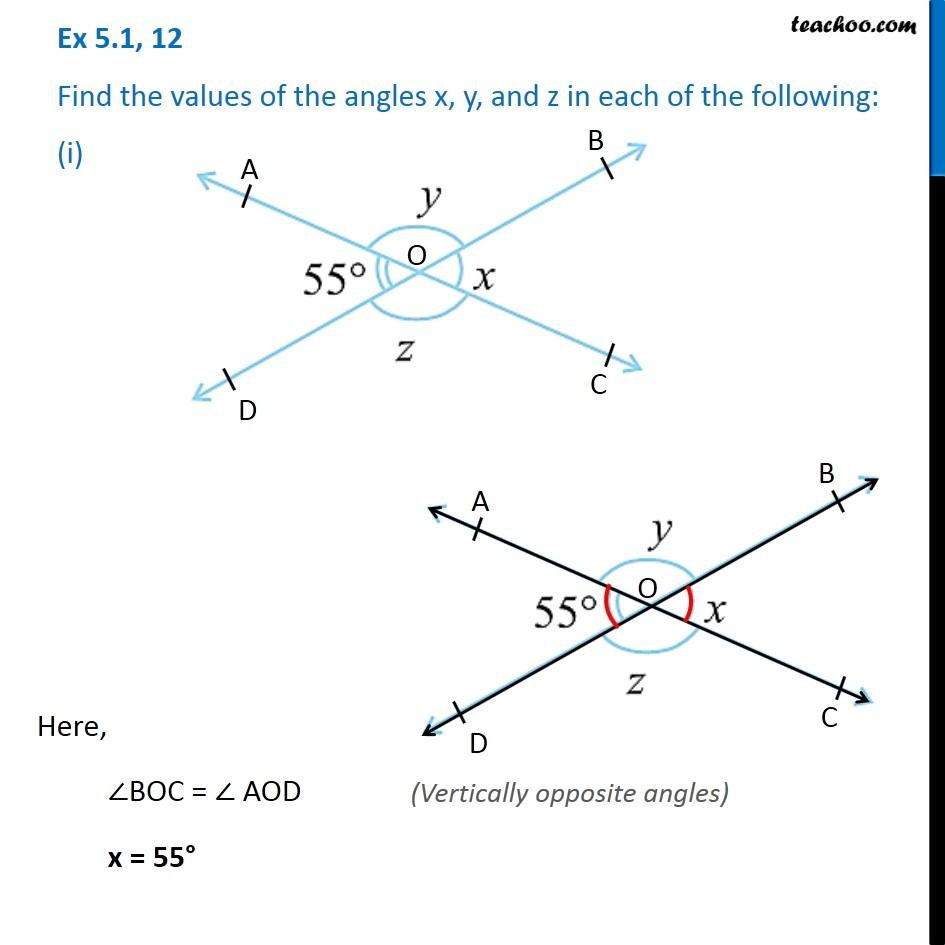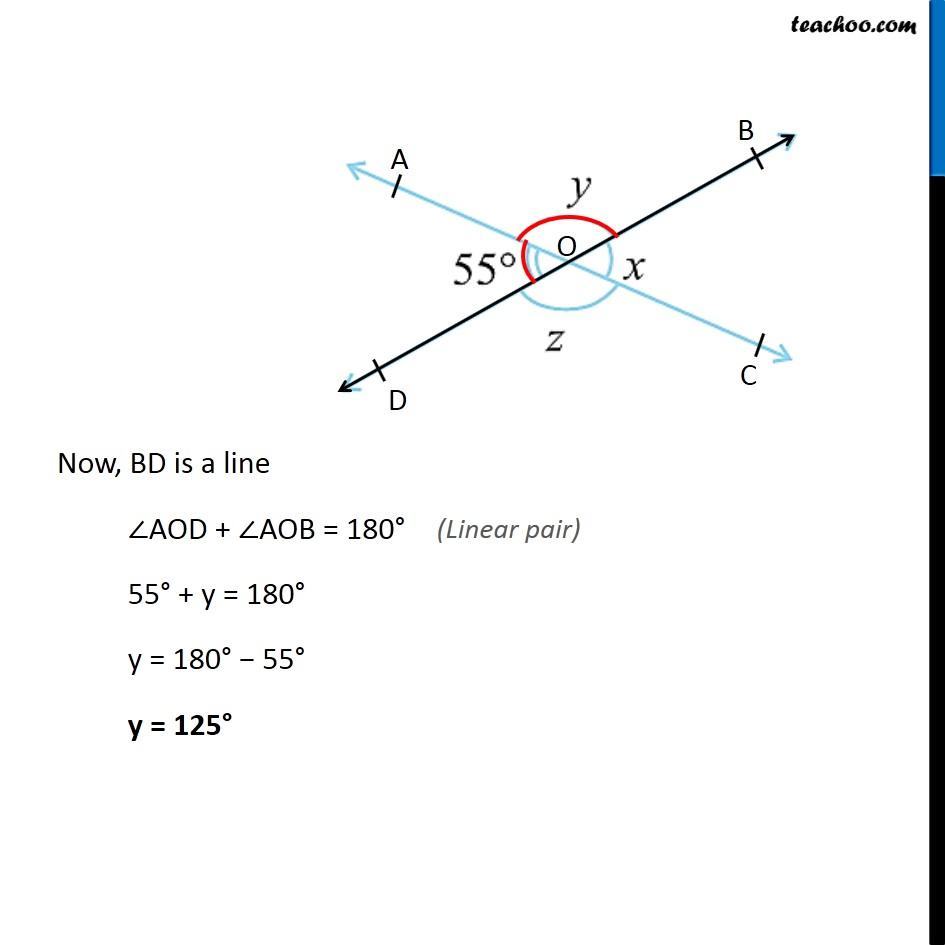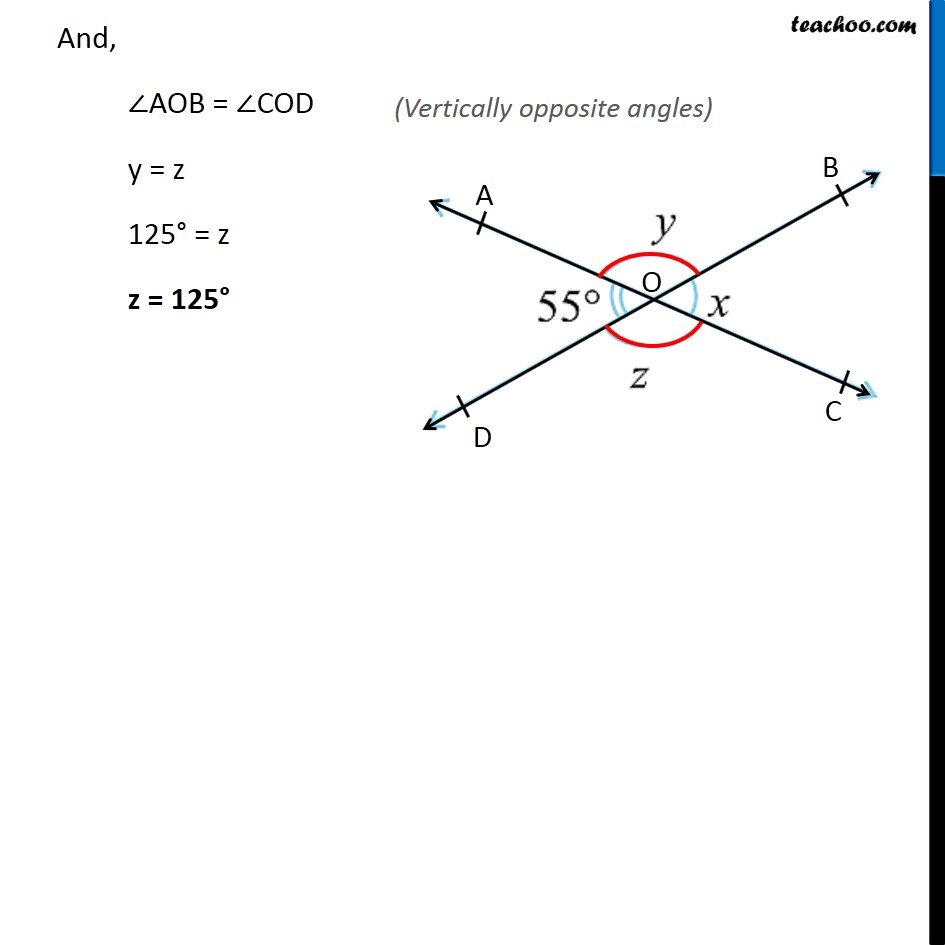1. Chapter 5 Class 7 Lines and Angles
2. Concept wise
3. Adjacent Angles, Linear Pair of angles, Vertically Opposite angles

Transcript

Ex 5.1, 12 Find the values of the angles x, y, and z in each of the following: (i)Ex 5.1, 12 Find the values of the angles x, y, and z in each of the following: (i)Vertically opposite angles) Vertically opposite angles) And, ∠AOB = ∠COD y = z 125° = z z = 125°

Adjacent Angles, Linear Pair of angles, Vertically Opposite angles